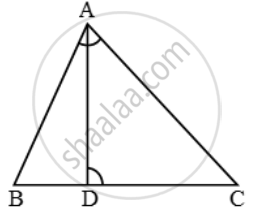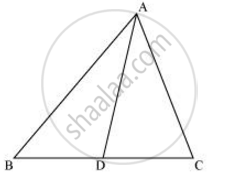Share

# D is a point on the side BC of ∆ABC such that ∠ADC = ∠BAC. Prove that CA/CD=CB/CAor, CA^2 = CB × CD. - CBSE Class 10 - Mathematics

ConceptCriteria for Similarity of Triangles

#### Question

D is a point on the side BC of ∆ABC such that ∠ADC = ∠BAC. Prove that  \frac{CA}{CD}=\frac{CB}{CA} or, CA^2 = CB × CD.

#### Solution 1

In ∆ABC and ∆DAC, we have

∠ADC = ∠BAC and ∠C = ∠CTherefore, by AA-criterion of similarity, we have

∆ABC ~ ∆DAC

\Rightarrow \frac{AB}{DA}=\frac{BC}{AC}=\frac{AC}{DC}

\Rightarrow \frac{CB}{CA}=\frac{CA}{CD}

#### Solution 2∠ACD = ∠BCA (Common angle)

∴ ΔADC ∼ ΔBAC (By AA similarity criterion)

We know that corresponding sides of similar triangles are in proportion.

:. (CA)/(CB) = (CD)/(CA)

=> CA2 = CB x CD

Is there an error in this question or solution?

#### APPEARS IN

NCERT Solution for Mathematics Textbook for Class 10 (2019 to Current)
Chapter 6: Triangles
Ex. 6.30 | Q: 13 | Page no. 141

#### Video TutorialsVIEW ALL 

Solution D is a point on the side BC of ∆ABC such that ∠ADC = ∠BAC. Prove that CA/CD=CB/CAor, CA^2 = CB × CD. Concept: Criteria for Similarity of Triangles.
S